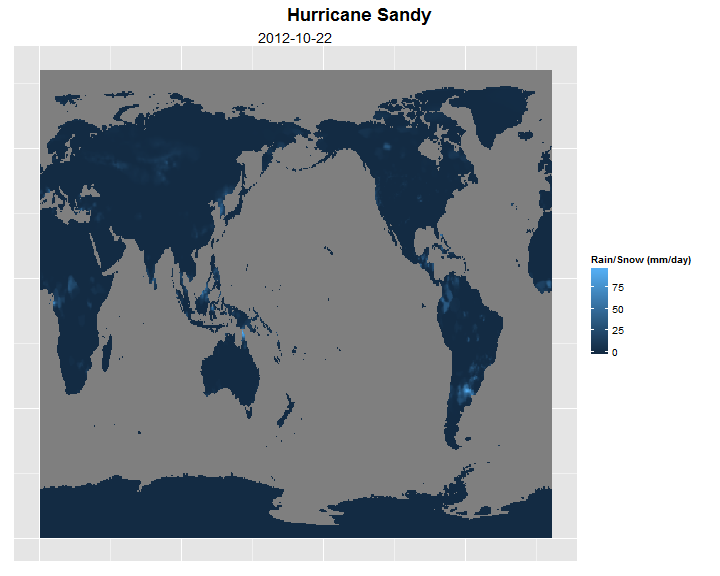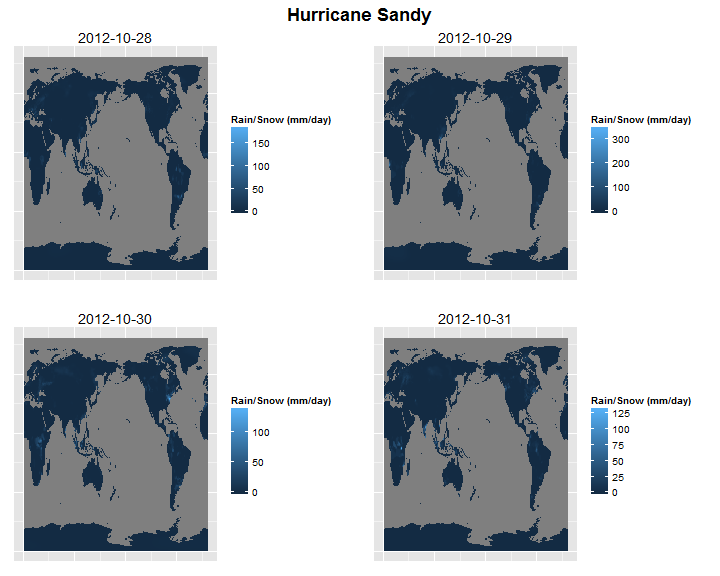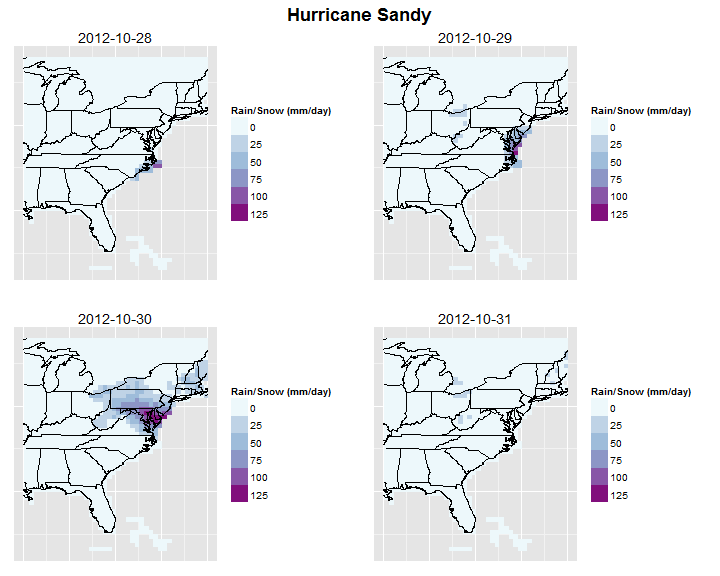# R code to obtain and plot rainfall data for the whole world

If you want to create rainfall maps for the whole world in R there is no readily available code or package to do this. Moreover, data publicly available from research institutions is not generally in plain text format. Hydrological and climatological studies sometimes require rainfall data over the entire world for long periods of time. The Climate Prediction Center's (CPC) site, daily data from 1979 to present, is a good resource. This data is available at CPC's ftp site (ftp://ftp.cpc.ncep.noaa.gov/precip/CPC_UNI_PRCP/GAUGE_GLB/).

I created R code to download rain/snow (or precipitation to be scientific) data from the CPC's ftp site and plot it. All my code is available at my GitHub site - https://github.com/RationShop/cpcRain.

Following examples demonstrate some of the things one could accomplish using my R code.

First, invoke the library of functions used to automate the file download process and also invoke libraries used for reformatting and plotting.

``````source("cpc_lib.R")

library(ggplot2)
library(reshape2)
library(gridExtra)  # for plotting multiple panels within a page
``````
``````## Loading required package: grid
``````

## Data for just one day - global plot

Example showing data download for a single day - 2012/10/22, the inception of Hurricane Sandy.

``````yr <- 2012
mo <- 10
day <- 22
# get raw data from CPC
# convert raw data to binary
# re-orient data for plotting
sandy <- Fn_Rotate_Matrix(Fn_Flip_Matrix_Rows(sandy))
# plot data
plot1 <- ggplot(data = melt(sandy)) + geom_raster(aes(Var1, Var2, fill = value)) +
theme(axis.text = element_blank(), axis.ticks = element_blank()) + labs(x = NULL,
y = NULL, fill = "Rain/Snow (mm/day)") + ggtitle(as.Date(paste(yr, mo, day,
sep = "-")))
# save plot
png("sandy1.png", width = 10, height = 8, units = "in", res = 72)
grid.arrange(arrangeGrob(plot1, main = textGrob("Hurricane Sandy", vjust = 1,
gp = gpar(fontface = "bold", cex = 1.5))))
garbage <- dev.off()
``````You could see that the Caribbean, where Sandy was on Oct 22nd, has a lot of rain. To answer my question in the title of this blog - it was also pouring in several other parts of the world (mainly tropical regions).

## Data for many days - global plot

If you want to download data for more than a day, or all the 34+ years of data (~ 365 * 34 files, it takes a while!) then here is another example. I download data for the duration of Sandy and then make a four-panel plot for four selected days.

``````# get raw data from CPC
# process and plot rain on 4 select days
days <- c(28, 29, 30, 31)
plot4 <- list()  # store ggplot output
for (eachDay in 1:length(days)) {
# convert raw data to binary
# re-orient data for plotting
sandy <- Fn_Rotate_Matrix(Fn_Flip_Matrix_Rows(sandy))
# plot data
plot4[[eachDay]] <- ggplot(data = melt(sandy)) + geom_raster(aes(Var1, Var2,
fill = value)) + theme(axis.text = element_blank(), axis.ticks = element_blank()) +
labs(x = NULL, y = NULL, fill = "Rain/Snow (mm/day)") + ggtitle(as.Date(paste(yr,
mo, days[eachDay], sep = "-")))
}
# save plot
png("sandy2.png", width = 10, height = 8, units = "in", res = 72)
grid.arrange(arrangeGrob(plot4[], plot4[], plot4[], plot4[], ncol = 2,
main = textGrob("Hurricane Sandy", vjust = 1, gp = gpar(fontface = "bold",
cex = 1.5))))
garbage <- dev.off()
``````This gives you more information than the previous plot, but it is not clear as to what is happening in any one region. You could kind of see that the hurricane is moving from the Caribbean towards the mid Atlantic coast.

## Data for many days - regional plot

In the next example, I plot only the eastern portion of the United States. I also cleaned up the plot to make the color scale consistent between the plots and to add state boundaries.

``````# lat-lon bounds of eastern US
maxLat <- 48
minLat <- 23
maxLon <- -70
minLon <- -90

# US state boundaries used by ggplot
boundaryState <- map_data("state")

# process and plot rain on 4 select days
days <- c(28, 29, 30, 31)
plot4 <- list()  # store ggplot output
for (eachDay in 1:length(days)) {
# convert raw data to binary
# extract regional data
sandy <- Fn_Get_Region_Data_For_Plot(sandy, maxLat, minLat, maxLon, minLon)
# plot data
plot4[[eachDay]] <- ggplot(data = sandy) + geom_raster(aes(lon, lat, fill = colorScale)) +
scale_fill_brewer(palette = "BuPu", drop = FALSE) + xlim(c(minLon, maxLon)) +
ylim(c(minLat, maxLat)) + geom_path(data = boundaryState, aes(x = long,
y = lat, group = group), na.rm = TRUE) + theme(axis.text = element_blank(),
axis.ticks = element_blank()) + labs(x = NULL, y = NULL, fill = "Rain/Snow (mm/day)") +
ggtitle(as.Date(paste(yr, mo, days[eachDay], sep = "-")))
}
# save plot
png("sandy3.png", width = 10, height = 8, units = "in", res = 72)
grid.arrange(arrangeGrob(plot4[], plot4[], plot4[], plot4[], ncol = 2,
main = textGrob("Hurricane Sandy", vjust = 1, gp = gpar(fontface = "bold",
cex = 1.5))))
garbage <- dev.off()
``````Now you can see the blob of heavy rain over New York - New Jersey area.

## Summary

Using this code a lot more analyses could be done. Hopefully you will find this code useful.